• Meet Marshall

### More Programs

• Marshall Life

• Athletics

## Faculty Biographies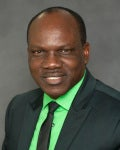Alfred Akinsete, Ph.D.
(Mathematical Statistics)

Dr. Akinsete’s research interests are in stochastic processes, applied probability, and distribution functions. He is currently interested in generating statistical distributions and their applications to real-life data. His other areas of research focuses on queuing networks, and the statistical analyses of sports data.

Email: akinsete@marshall.edu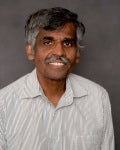Ari Aluthge, Ph.D.
Vanderbilt University
(Functional Analysis, Operator Theory)

Dr. Aluthge’s research interest includes p-hyponormal operators and operator inequalities. He is mainly interested in properties of p-hyponormal operators. His recent research involves results related to spectral properties.

Email: aluthge@marshall.eduRaid Al-Aqtash, Ph.D.
Central Michigan University
(Statistical Distributions Theory)

Dr. Al-Aqtash’s research focuses on generating new families of T-X{Y} distributions by combining two existing distributions using a suitable link transformation, studying their mathematical properties and application to different real life phenomena. Other research interests include pattern recognition, data mining, time series, and regression modelling.Andrea Duhon, Ph.D.
Columbia University
(Language in Mathematics, Mathematics Education)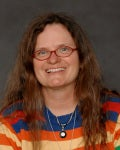Bonita Lawrence, Ph.D.
University of Texas, Arlington
(Dynamical Systems)

Dr. Lawrence’s research interests include calculus and analysis on time scales. This field generalizes discrete mathematics and continuous mathematics into a single system. Dr. Lawrence also heads the Marshall University Differential Analyzer Lab. This lab builds differential analyzers, which are analog machines for solving differential equations, and uses them to study differential equations and time scales calculus.Avishek Mallick, Ph.D.
University of Louisiana at Lafayette
(Statistics)

Dr. Mallick’s interests are distribution theory and statistical modeling, especially developing statistical methodologies for analyzing environmental and medical/biological data. Currently, his focus is analysis of censored survival data and its application in Medicine and Environmental Sciences. He is also working on developing inferential procedures for discrete inflated distributions and their applications. His other research interests include missing data analysis, especially multiple imputation and statistical meta-analysis.Anna Mummert, Ph.D.
Pennsylvania State University
(Dynamical Systems, Mathematical Biology)

Dr. Mummert’s research interests are in Epidemiological and Ecological Modeling (Mathematical Biology) and Dynamical Systems. Currently, she is involved in studying the inverse problem of recovering the time-dependent transmission rate of an epidemiological model given incidence data in stochastic differential equations, the prairie ecoregion, questions around fresh drinking water in Iowa and WV, and using dynamical systems tools to understand mathematical biology models.Carl Mummert, Ph.D.
Pennsylvania State University (Mathematical Logic)

Dr. Mummert’s research is in mathematical logic, particularly in reverse mathematics, an area of logic seeks to determine which axioms are necessary to prove well-known theorems of mathematics. His reverse mathematics research has focused on principles from topology, choice principles, and countable combinatorics. He has also published papers in pure topology, and has mentored student research in logic, real analysis, and latin squares.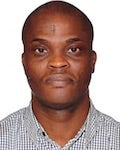Michael Otunuga, Ph.D.
University of South Florida
(Dynamical Systems and Stochastic Dynamical Modeling)

Dr. Otunuga’s research interests include Stochastic Dynamical Systems and Analysis; Mathematical Biology (Deterministic and Stochastic Dynamics of Infectious Diseases); and Commodities Price Modeling and Analysis. Currently, he is involved in studying the global dynamics of multi-dimensional epidemic models with treatments. He is also focusing on a time varying parameter estimation scheme for a stochastic D.E. and modeling and analysis of financial data (energy commodity, U.S. Treasury Interest rate, U.S.-U.K. exchange rate, stock price data, etc.)

Email: otunuga@marshall.edu

Webpage: http://science.marshall.edu/otunuga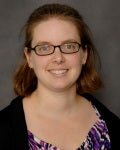Elizabeth Niese, Ph.D.
Virginia Polytechnic Institute and State University
(Algebraic Combinatorics)

Dr. Niese studies symmetric and quasisymmetric functions. These functions have many connections to both algebra and combinatorics. Dr. Niese’s current research includes working on generalizations of quasisymmetric Schur functions and combinatorial properties of Macdonald polynomials.

Email: niese@marshall.eduScott Sarra, Ph.D.
West Virginia University
(Numerical Analysis)

Dr. Sarra is an active researcher in numerical analysis. His research is with high-order methods for the numerical solution of partial differential equations (PDEs). The methods include radial basis function methods (RBFs) and pseudospectral methods. He is also involved with post-processing methods that reduce or eliminate the Gibbs phenomenon.

Email: sarra@marshall.edu

Webpage: http://www.scottsarra.org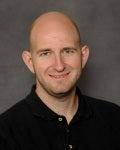Michael Schroeder, Ph.D.